KERNEL METHOD - Web Application - Optimization Method - Original paper - Presentation - FAQ - Code Links - Histogram Optimization - Kernel Optimization - State-space analysis - Point process - HOME Page

 Kernel Bandwidth Optimization Web application for optimizing kernel density estimate

 Shimazaki and Shinomoto. J Comput Neurosci, 2010, 29 (1-2) 171-182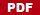## Optimize Density Profile of Your Data

 Web Application for Kernel Bandwidth Optimization (Ver. 0.4) 1. Copy&Paste your data 4.37 3.87 4.00 4.03 3.50 4.08 2.25 4.70 1.73 4.93 1.73 4.62 3.43 4.25 1.68 3.92 3.68 3.10 4.03 1.77 4.08 1.75 3.20 1.85 4.62 1.97 4.50 3.92 4.35 2.33 3.83 1.88 4.60 1.80 4.73 1.77 4.57 1.85 3.52 4.00 3.70 3.72 4.25 3.58 3.80 3.77 3.75 2.50 4.50 4.10 3.70 3.80 3.43 4.00 2.27 4.40 4.05 4.25 3.33 2.00 4.33 2.93 4.58 1.90 3.58 3.73 3.73 1.82 4.63 3.50 4.00 3.67 1.67 4.60 1.67 4.00 1.80 4.42 1.90 4.63 2.93 3.50 1.97 4.28 1.83 4.13 1.83 4.65 4.20 3.93 4.33 1.83 4.53 2.03 4.18 4.43 4.07 4.13 3.95 4.10 2.72 4.58 1.90 4.50 1.95 4.83 4.12 2. 3. * The Gauss kernel density was used. Its standard deviation is optmized. * Feel safe. The data is processed on your own computer (NEVER transferred to our server). * This web application uses the HTML5 Canvas technology.ver 0.4 (101231) accelarating computation by excluding >5 std samples. Web Application for Kernel Bandwidth Optimization © 2009-2011Hideaki Shimazaki

## Kernel Bandwidth Optimization Method

 I. Letbe your data. Let the kernel with bandwidthbe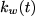. II. Compute a formula,III. Find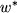that minimizes. From the observed data only, the method estimates a bandwidth that minimizes expected L2 loss between the kernel estimate and an unknown underlying density function. An assumption made here is merely that samples are drawn from the density independently each other.

## Locally Adaptive Kernel Bandwidth Optimization Method

 In the referenced paper, we developed a locally adaptive bandwidth optimization method. The locally adaptive bandwidth is obtained by iteratively computing optimal bandwidths wihtihn local intervals using the algorithm above. In this approach, the local interval length is a critical parameter that determines the smoothness of bandwidth, and therefore goodness-of-fit of the density estimate. We suggested, at every points of estimation, to select the interval length such that the locally optimal bandwidth covers \gamma *100 % of the interval length (0<\gamma<=1). In other words, the selected bandwidth, w, is optimal as evaluated within the local interval of the length w / \gamma . The parameter, \gamma, serves as a smoothness (or stiffness) parameter of the variable bandwidth just like the bandwidth is the smoothness parameter of the estimated density. The stiffness constant, \gamma, is optimized by minimizing the L2 risk estimate. Example: An underlying densityi is a mixture of two normal densities and an exponential density.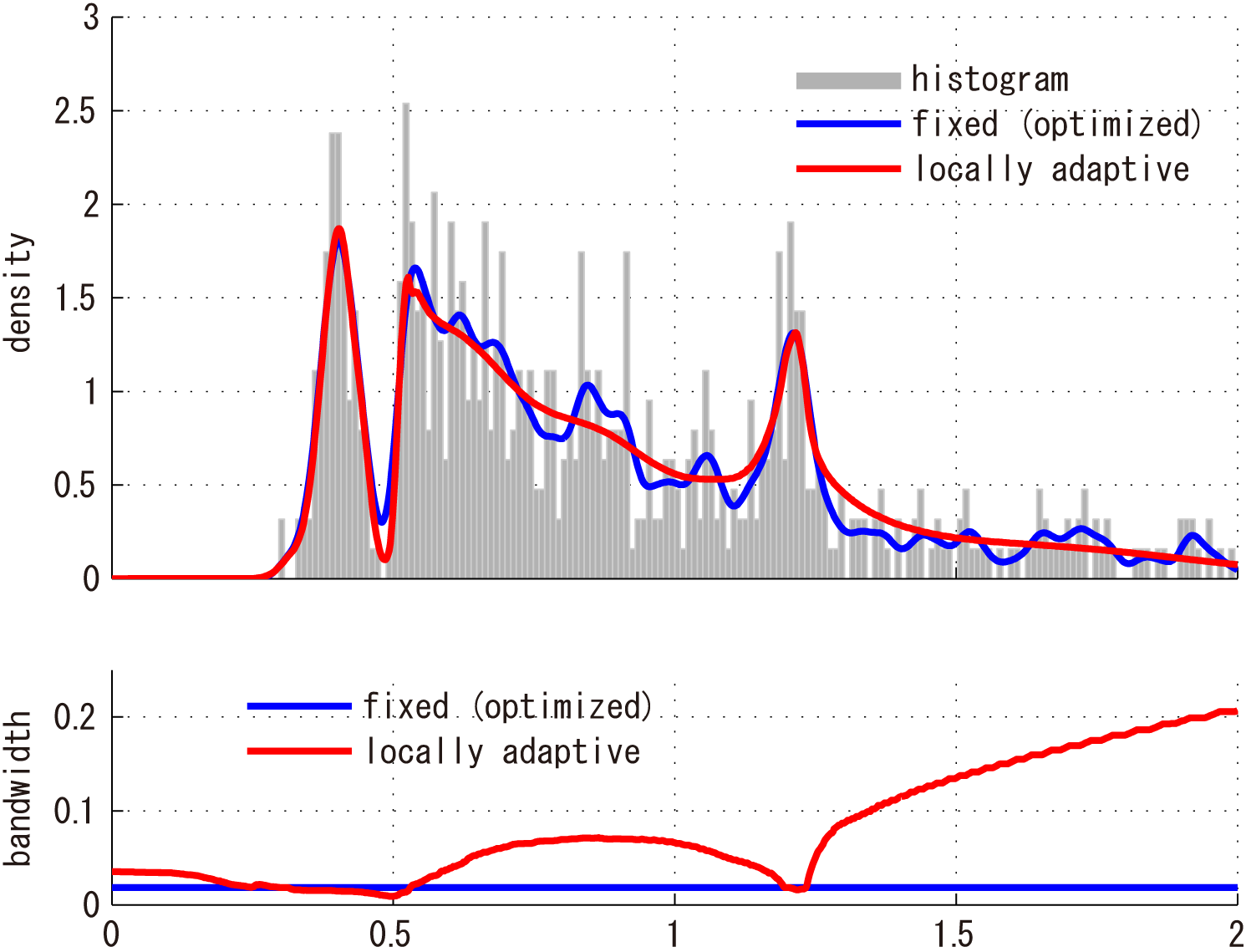' A Matlab code for locally adaptive kernel method is available below.

### Original paperShimazaki H. and Shinomoto S., Kernel Bandwidth Optimization in Spike Rate Estimation. Journal of Computational Neuroscience (2010) Vol. 29 (1-2) 171-182 doi:10.1007/s10827-009-0180-4 [Open Access: PDF, LINK]Presentation Slides

### The method has been used in a variety of fields in Science

 The method has been used in a variety of scientific fields. Example usages in Neuroscience are found at Hill, M.R., Fried, I. and Koch, C., 2014. Quantification and classification of neuronal responses in kernel-smoothed peristimulus time histograms. Journal of neurophysiology, 113(4), pp.1260-1274. J Neurophysiol2015. Ito, H.T., Zhang, S.J., Witter, M.P., Moser, E.I. and Moser, M.B., 2015. A prefrontal–thalamo–hippocampal circuit for goal-directed spatial navigation. Nature, 522(7554), p.50. Nature 2015. Manita, S., Suzuki, T., Homma, C., Matsumoto, T., Odagawa, M., Yamada, K., Ota, K., Matsubara, C., Inutsuka, A., Sato, M. and Ohkura, M., 2015. A top-down cortical circuit for accurate sensory perception. Neuron, 86(5), pp.1304-1316. Neuron 2015.

## Matlab Code

 Kernel density estimation by a fixed bandwidth Matlab code: sskernel.m Function sskernel' returns optimal bandwidth (standard deviation) of the Gauss kernel function used in kernel density estimation. Locally adaptive kernel density estimation Matlab code: ssvkernel.m Function ssvkernel' returns an optimized kernel density estimate using a Gauss kernel function with bandwidths locally adapted to the data. tutorial.mThe codes are available at GitHub. http://github.com/shimazaki/density_estimation Experimental Julia code: here.

## Python Code (Contribution by Lee Cooper and Subhasis Ray)

 Lee Cooper created python program of kernel and histogram optimization. This is an extended version of the translation by Subhasis Ray. - 2016/5/6 The adaptive kernel density estimation module is can be installed by typing pip adaptivekde See also

 pdf

## FAQ

 Q. Is the method applicable to probability density estimation? A. Yes. Q. You provide a histogram optimization method, too. Which of the methods, kernel and histogram, do you recommend for density estimation. A. Kernel density estimation is generally recommended. See our original paper for comparison of the methods. Q. Is it different from the least squares cross-validation method? A. Yes. The above formula was derrived under a Poisson point process assumption [see our original paper], not by the cross-validation. As a result, chosen bandwidths by the two methods are not identical. Q. Can I use the method for the 2-dimensional density estimation? A. Yes. The same optimization formula can be used in 2-d density estimation. Specifically, the formula for two dimensional vector data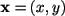is given by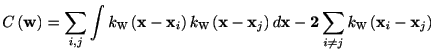Here the kernel function is defined in 2-dimension. For example, the 2-d (symmetric) Gauss kernel is defined aswhere. An example of the optimized 2d kernel density estimate is displayed below.Here are the example matlab code sskernel2d_demo.m and data. Note: if you have two dimensional variables with different dynamic ranges, it should be careful to use the one-parameter 2-d kernel. It would be recommended to either i) optimize two-parameters kernel, or ii) optimize the one-parameter kernel using the standardized data, for example, by dividing the data by standard deviations of each component. Q. Can I compare the goodness-of-fit of kernel density estimate with that of a histogram? The definition of the cost function is different in the two papers (2007 and 2010). To compare the cost function of a histogram with the cost function of a kernel, please adjust the histogram cost function as T*C(D)+K^2/T, where K is the total number of samples, and T is the observation length.   The FAQ includes my (HS) opinion. They are not opinions neither by my collaborators nor institutions I belong to.